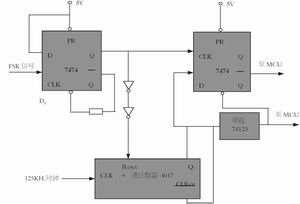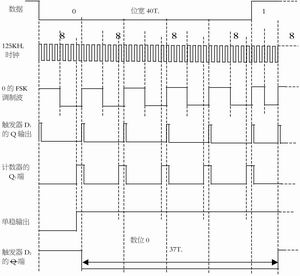# Design of FSK Demodulator for 125KHz RFID Reader

PickÂ Â  Important : This article provides an FSK demodulator circuit for 125KHz non-contact RFID readers , which can demodulate FSK signals into NRZ codes. This circuit is simple and practical, and can be used for various FSK modulation modes set in RFID chips. demodulation.

introduction

Many RFID chips that work at 125KHz carrier frequency , such as Microchip 's MCRF200 and MCRF250, and Atmel 's e5551 , T5557 , etc., can set their modulation mode to FSK . If the chip is set to FSK modulation mode, then the reader/writer (PCD) must have an FSK demodulation circuit. The FSK demodulation circuit demodulates the FSK modulated signal into an NRZ code.

This text provides a kind of FSK demodulation circuit, the characteristic of this circuit is that the circuit is simple and reliable, very suitable for the application in PCD .

FSK modulation

The FSK modulation methods of RFID working at 125KHz are very similar. Figure 1 shows a waveform diagram of an FSK modulation method. It can be seen from the figure that the data rate at this time is: carrier frequency fc/40=125K/40=3125bps , after FSK modulation, data 0 is a square wave with a frequency of fc/8 , that is, f0= fc/8 ; and data 1 is a square wave with a frequency of fc/5 , that is, f1= fc/5 .

The transmission data after FSK modulation is transmitted to the PCD through the load modulation method . The radio frequency waveform is also shown in Figure 1. The modulation of the carrier is amplitude modulation.

FSK demodulation

After the PCD undergoes carrier demodulation ( usually using envelope detection ) , amplifying filtering and pulse shaping circuit, the FSK modulated signal is obtained . The FSK demodulation circuit completes the restoration of the FSK modulated signal to the NRZ code. There are many ways to implement FSK demodulation. An FSK demodulation circuit introduced in this article is shown in Figure 2. This circuit is simple and convenient, and can well complete FSK demodulation.

The working principle of the circuit shown in Figure 2 is as follows: flip-flop D1 turns the input FSK signal into a narrow pulse, that is, when Q is high, the upper edge of FSK sets Q terminal high, but since Q is low at this time , CL terminal is low , And make the Q terminal return to low level. This pulse on the Q terminal resets the decimal counter 4017 and recounts .

The 4017 counter counts the 125KHz clock . Since the data width is 40/fc=40Tc (Tc is the carrier cycle ) , if it is data 0 , the FSK square wave cycle T0=8Tc . When the 7th clock is counted, the output of Q7 is high, making CLKen (CLK enable terminal ) high, and the counter no longer counts the 8th clock. At this time, Q7 is high. When the Q output terminal of flip-flop D1 is down When an FSK waveform jumps up, the Q terminal output of flip-flop D2 is low. Jumping on the FSK waveform also resets the counter to zero and recounts. Thus, the FSK data corresponding to the frequency of the waveform of 0, Q output of the flip-flop D2 is low, that is, data 0 of the NRZ code level.Picture 2Â  FSK demodulation circuitFigure 3Â  Example of demodulated waveform of digit 0 ( followed by bit 1)

When the data 1, since the FSK waveform period T1 = 5Tc, so the pin Q7 of counter 4017 is always low, during which the Q output of the flip-flop D2 is kept high, that is, NRZ data level one.

The demodulated waveform of data 0 is shown in Figure 3 . Seen from the figure, if the bit 0 is followed 0, then the bit width of 40Tc, followed if the bit is 1, the bit width which is 37Tc, short three clock cycles. The following bit of bit 1 is 1 , and its bit width remains 40Tc. If the following bit is 0 , its bit width is 43Tc . Therefore, the interleaving of bit values 0 and 1 does not cause the propagation of bit width errors, but compensates. Â± 3 clock errors, will not affect the accuracy of the MCU positioning judgment.

The up-jump change generated by the monostable circuit provides the CL terminal level of the flip-flop D2 with normal operation , and also informs the MCU that the output data of the flip-flop D2 is valid after that. The monostable circuit can be 74HC123 , which is a repeatable trigger monostable circuit that can automatically start and close the demodulator.

RFID chip FSK usually have multiple modes, such as in one of four modes e5551 (Table 1), the analysis of the circuit described above corresponds FSK1a, but FSK1, just to the output terminal Q of the flip-flop D2 That's it. If FSK2 is used , the output terminal of the counter can be changed to Q9 .

For different data rates, only the bit width is different, which does not affect the demodulation result.

Concluding remarks

The circuit is simple and reliable, and has been used in water meter reading heads.

Phenolic Paper Laminated Sheet

Phenolic paper laminated sheet is a hard, dense material made by applying heat and pressure to layers of paper or fabric, impregnated with a synthetic resin. These phenolics are approx half the weight of aluminum and their physical toughness resists abrasion, friction, impact, and material fatigue. Special properties fight off the corrosive action of water and many chemicals, increasing the service life and efficiency of your product parts. The insulating qualities of each grade, such as low dissipation factor and excellent dielectric strength, make them valuable for many precise electrical and electronic applications.
It is made of insulating impregnated paper impregnated with phenolic resin and baked and hot pressed. Has high mechanical properties. The heat resistance level is E level.

Phenolic Paper,Phenolic Cardboard,Paper Phenolic Sheet,Phenolic Paper Laminated Sheet

Yingkou Dongyuan Electrical Insulation Board Co.,Ltd , https://www.dy-insulation.com